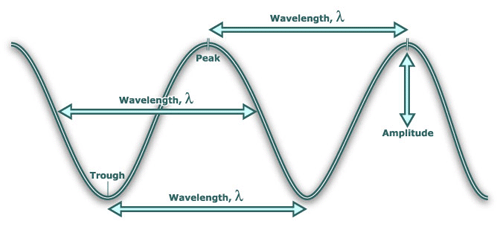In physics wavelength is the distance between repeating units of a propagating wave of a given frequency. It is commonly designated by the Greek letter lambda (λ). Examples of wave-like phenomena are light, water waves, and sound waves. The wavelength is related to the frequency by the formula: wavelength = wave speed / frequency. Wavelength is therefore inversely proportional to frequency. Higher frequencies have shorter wavelengths. Lower frequencies have longer wavelengths, assuming the speed of the wave is the same.

In a wave, properties vary with position. For example, in a sound wave the air pressure oscillates, while in light and other electromagnetic radiation the strength of the electric and the magnetic field vary.

Visible light ranges from deep red, roughly 700 nm, to violet, roughly 400 nm (430–750 THz). For other examples, see electromagnetic spectrum. The wavelengths of sound frequencies audible to the human ear (20 Hz–20 kHz) are between approximately 17 m and 17 mm, respectively. So the wavelengths in audible sound are much longer than those in visible light.

Examples of wave-like phenomena are sound waves, light, and water waves. A sound wave is a periodic variation in air pressure, while in light and other electromagnetic radiation the strength of the electric and the magnetic field vary. Water waves are periodic variations in the height of a body of water. In a crystal lattice vibration, atomic positions vary periodically in both lattice position and time.

Wavelength is a measure of the distance between repetitions of a shape feature such as peaks, valleys, or zero-crossings, not a measure of how far any given particle moves. For example, in waves over deep water a particle in the water moves in a circle of the same diameter as the wave height, unrelated to wavelength.

Speed of wave, v = frequency, f x wavelength, λ